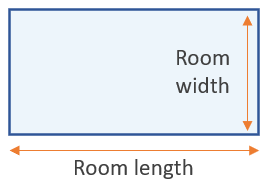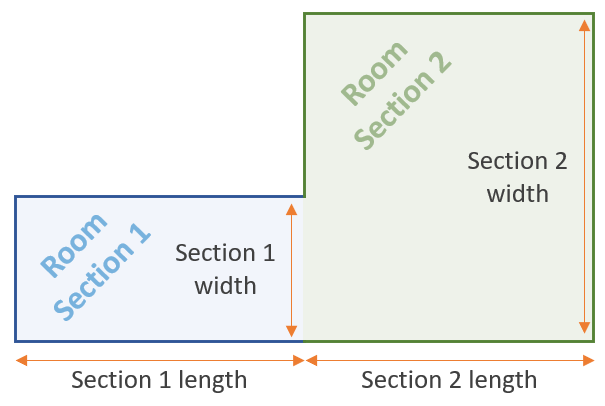# Square Footage Calculator

Use this square footage calculator to easily calculate the area of a rectangular room or a room with a more complex shape. Room size calculation in square feet. Optional price field for calculating dollar costs. Formulas and explanation below.

\$
Share calculator:

Embed this tool:
get code

## How to calculate square footage

Square footage is a measurement of the area of a room (or other type of space) expressed in feet square (ft2). While each geometrical figure has a different formula for calculating its area, most rooms have an approximately rectangular shape. Therefore, if you can measure the width and length of the room, you can easily calculate the square footage by simply multiplying the two measurements.

The formula for the area of a rectangular room is width x length, as shown in the figure below:This is the equation used in our square footage calculator as well. Note that if you measure the room in inches, the result will be in square inches. If you measure it in yards, it will be in square yards, and so on with metric units such as centimeters and meters (results in sq cm and sq m respectively). To get a result in square feet, make sure the input measurements are in square feet as well (you can remember it as "ft to sq ft").

A rectangle room is one of the simplest types of area shapes for which square footage can be calculated. A square room will be even simpler since the length is equal to the width. For example, the size of a 10 ft by 10 ft room (10x10 room) is 100 sq ft (102). Likewise, a 15x15 room is 225 sq ft (152), while a 20x20 room is 400 sq ft (202). However, perfectly square spaces are rarely encountered outside of classroom tasks.

## Square footage for a room with a complex shape

In the real world most rooms deviate from a rectangle to an extent. There might be nooks or crannies, as well as protrusions, which if small, can be overlooked for simplicity since rounding and other considerations taken into account in a construction or internal decoration project usually have fairly large tolerances. However, it is common for a room to consist of two (usually) differently sized rectangles as shown in the figure below:If that is the case, then you need to take not two, but four measurements to figure out the square footage: the width and the height of the first rectangular section and then the width and the height of the second rectangular section of the room. The total area in square feet or another unit is just the sum of the areas of the two rectangles and you can calculate it easily using our tool.

If a room has a significantly irregular shape, then you would need to break it down into a series of rectangles, so that their square footage can be calculated approximately. The overall room square footage is then the sum of the smaller sections it was decomposed into. You can use this calculator to compute each room's size and our summation calculator to calculate their sum.

## From square footage to total price of a construction or decoration project

Now that we know how to calculate the square footage, we can turn to calculating the total price for a decoration or construction project. If you are laying down flooring, heating, tiling, gravel, mulch, etc. you might want to know how much it would cost you given the calculated footage. To arrive at the total cost, all you need to do is to know the price per square foot (or the square unit of your choice) and to then multiply it by the number of area units.

Say you have a complex-shaped room composed of a 10x10 area adjacent to a 12x24 area. The area of the first one is 100 ft2 and the area of the second is 288 ft2, so the total square footage is 100 + 288 = 388 square feet. Say it costs \$10 to cover one square foot in laminate flooring. To figure out the required cost for this room, multiply the calculated square footage by the cost per square foot: 388 x \$10, to get the total cost which is \$3,880 in this case. Such computations are handled by our calculator as well.

#### Cite this calculator & page

If you'd like to cite this online calculator resource and information as provided on the page, you can use the following citation:
Georgiev G.Z., "Square Footage Calculator", [online] Available at: https://www.gigacalculator.com/calculators/square-footage-calculator.php URL [Accessed Date: 01 Apr, 2023].